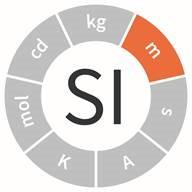An official website of the United States governmentOfficial websites use .gov
A .gov website belongs to an official government organization in the United States.Secure .gov websites use HTTPS
A lock ( ) or https:// means you've safely connected to the .gov website. Share sensitive information only on official, secure websites.

# SI Units - Length

## ShareThe meter (m) is defined by taking the fixed numerical value of the speed of light in vacuum c to be 299,792,458 when expressed in the unit m s−1, where the second is defined in terms of ∆νCs.

The meter was once defined by a physical artifact - two marks inscribed on a platinum-iridium bar. The Length - Evolution from Measurement Standard to a Fundamental Constant explains the evolution of the definition of the meter. Follow these changes over time in the NIST Length Timeline.

From the meter, several other units of measure are derived such as the:

• unit of speed is the meter per second (m/s). The speed of light in vacuum is 299 792 458 meters per second.
• unit of acceleration is the meter per second per second (m/s2).
• unit of area is the square meter (m2).
• unit of volume is the cubic meter (m3). The liter (1 cubic decimeter), although not an SI unit, is accepted for use with the SI and is commonly used to measure fluid volume.

### FAQ: When did the metric redefinition of the inch occur?

In 1958, a conference of English-speaking nations agreed to unify their standards of length and mass, and define them in terms of metric measures. The American yard was shortened and the imperial yard was lengthened as a result. The new conversion factors were announced in 1959 in Federal Register Notice 59-5442 (June 30, 1959), which states the definition of a standard inch: The value for the inch, derived from the value of the Yard effective July 1, 1959, is exactly equivalent to 25.4 mm.

The conversion factor can be determined:Units of Length 10 millimeters (mm) = 1 centimeter (cm) 10 centimeters = 1 decimeter (dm) 10 centimeters = 100 millimeters 10 decimeters = 1 meter (m) 10 decimeters = 1000 millimeters 10 meters = 1 dekameter (dam) 10 dekameters = 1 hectometer (hm) 10 dekameters = 100 meters 10 hectometers = 1 kilometer (km) 10 hectometers = 1000 meters

### FAQ: How do I get a metric ruler?

Metric rulers are available from many retail vendors, which can be identified by using search terms such as "metric rule," "meter stick," or "metric stick." Printable rulers, such as the centimeter Color-square rules, can be color printed on to overhead transparency sheets to make inexpensive metric rulers.

### Educational Resources

• NIST – National Prototype Meter No. 27
• Using a Metric Ruler (Video)
• Using a Micrometer (University of Toronto)
• Using a Caliper and Micrometer (University of Capetown, Department of Physics)
• Examine Cell Size and Scale using an interactive graphic (University of Utah)
• Practice measuring length with centimeters in the Squares and Rectangles activity. (PBS)
• Develop an understanding of how small a nanometer really is with the What is a Nanometer activity?  During the lesson students will measure common classroom objects and convert the results to nanometers. (IEEE)
•Become familiar with equivalent metric length measurements with the Length Column Game. Draw a line to connect between like measurements. Look carefully, because some items do not have a match! (Quintessential Instructional Archive)
• Solve a real life problem.  Design, plan, and draw a garden layout to scale using a metric ruler. (University of California at Berkley, University of Nottingham)

### League of SI Superheroes – Meter Man:

This comic book-style video animation series has been developed to help middle school students learn about the 7 SI base measurement units. With his sharp eyes and stretchy ruler arms, no distance is too big or small for Meter Man to measure. A meter is the distance light travels in a tiny fraction of a second.

## Contacts

• Metric Program
Created April 12, 2010, Updated September 14, 2020